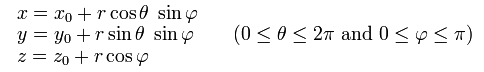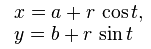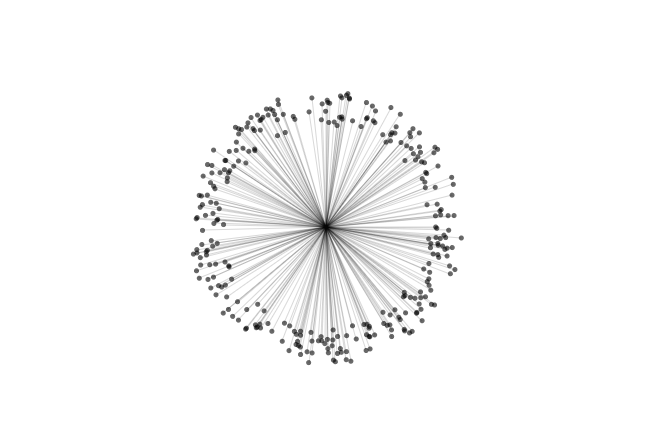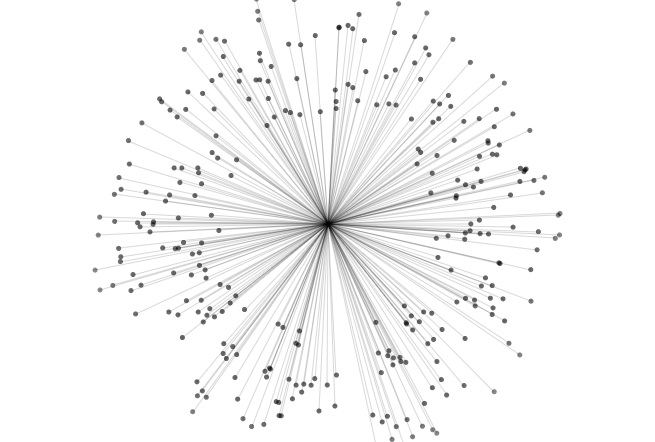# Audio visualizer

Inspiration (Magnetosphere, by Robert Hodgin):

https://vimeo.com/8581392

I believe this was not supposed to be a tutorial or anything, but some people were curious to know how I did this exercise. Here’s how:

I started this sketch in order to study some behaviors Iâ€™ve seen on Robert Hodginâ€™s work (see videoÂ above). The idea was not so much to copy but to try to practice the skills necessary to achieve a specific result. In this case using a mathematical formula to place points around a sphere (check this nerdy post for more details â€” that is, if you can handle :D ):The basic formula for the sphere in computer parlance looks like this:

x = cos( separationAngle * 2 * (PI/180) * theta) * sin( separationAngle * (PI/180) * fi ) * radius;
y = sin( separationAngle * 2 * (PI/180) * theta) * sin( separationAngle * (PI/180) * fi ) * radius;
z = cos( separationAngle * (PI/180) * fi ) * radius;

separationAngle is the angle between 2 successive points (360/numberOfPoints; more points => smaller angle
PI/180 converts degrees to radians (case youâ€™re wondering)

For simplicityâ€™s sake I started with the two-dimensional version of the problem, a circle:I created a Processing sketch to illustrate this (code for this is given at the bottom of the post):Next step was to make each point float in an interesting way. This was achieved by adding a value to the radius, relative to the position of the point in the sphere and a variation to the separationAngle:

x = cos(theta*(separationAngle + ang)*(PI/180)) * sin(fi*((separationAngle + ang)/2)*(PI/180)) * (radius + radVariation);
y = sin(theta*(separationAngle + ang)*(PI/180)) * sin(fi*((separationAngle + ang)/2)*(PI/180)) * (radius + radVariation);

ang ads some randomness to the separationAngle at the hit of UP or DOWN keyboard keys

cos( 1 * (PI/180) + (theta/4 + waveTravel) ) *
sin( 1 * (PI/180) + (fi/4 + waveTravel) ) *

spread is the amount of variation added to the radius at each point. it gets a boost every time the mouse is pressed or some loudness is detected in the input

And that’s about it for now!

================================================

Processing version (you should be able to run this on your Processing-ready computer; it also works in JS mode \o/ â€” bless these nerds, also you may recognize some variable names from this nice fellow’s post from elsewhere):

```int bgcolor = 255;
int numParticles = 300;```
```float radius;
float speed;
float angSpeed;
float gravity;
float friction;
float angRand[] = new float[numParticles];```
```void setup(){
background(bgcolor);
size(700, 500);

speed = 0;
angSpeed = 0;
gravity = 0.00001;
friction = .9999;
```for(int i = 0; i < numParticles; i++){
angRand[i] = random(-180, 180);
}
}```
```void draw(){
background(bgcolor);

translate(width/2, height/2);

for(int i = 0; i < numParticles; i++){

// Accelerate (change speed) by gravity
speed += gravity;
// Move by speed

// Bounce off Circle
// Reverse direction
speed *= -1;
}
speed *= friction;

angSpeed += gravity;
angSpeed *= friction;

stroke(0, 20);
line(x, y, 0, 0);
noStroke();
fill(0, constrain(255 - radiusIncr, 0, 150) );
ellipse(x, y, 5, 5);
}
}```
```void mousePressed(){
speed -= .1;
angSpeed -= 2;
}```
This entry was posted in Multimedia Authoring 2013-12, openFrameworks, Processing. Bookmark the permalink.

### One Response to Audio visualizer

1. Pingback: myGrain | RÃ©gis Frias